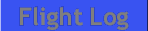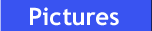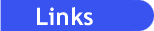#!/usr/bin/perl use CGI; #For CGI processing use DBI; #For Database access #Define the CGI Query and print header \$query = CGI::new(); print \$query->header; #Set the database parameters \$user = "nouser"; \$password = "nopassword"; \$dsn = "DBI:mysql:database=flying;host=deadhost"; \$dbh = DBI->connect(\$dsn, \$user, \$password); \$table = "flight"; \$statement="select * from flight"; \$sth=\$dbh->prepare(\$statement) or die "Can't execute \$statement: \$dbh->errstr\n"; \$rv = \$sth->execute or die "can't execute query: \$sth->errstr\n"; \$total_day_landings=0; \$total_night_landings=0; \$total_night_time=0; \$total_training_time=0; \$total_solo_time =0; \$total_xc_time =0; \$total_hood_time =0; \$total_time=0; #Display flight Summary print "AC's Flight Log"; print <<"EOF";EOF print "

# Flight Summary

"; print ""; while(\$hash_ref = \$sth->fetchrow_hashref){ print ""; print ""; print "\n"; print ""; print ""; print "\n"; print ""; print ""; print ""; print ""; print ""; print ""; print ""; \$total_day_landings = \$total_day_landings + \$hash_ref->{dayland}; \$total_night_landings = \$total_night_landings + \$hash_ref->{nightland}; \$total_night_time = \$total_night_time + \$hash_ref->{hrs_night}; \$total_training_time = \$total_training_time + \$hash_ref->{hrs_training}; \$total_solo_time = \$total_solo_time + \$hash_ref->{hrs_pic}; \$total_xc_time = \$total_xc_time + \$hash_ref->{hrs_xc}; \$total_hood_time = \$total_hood_time + \$hash_ref->{hrs_hood}; \$total_time = \$total_time + \$hash_ref->{hrs_total}; } print "
Date A/C Type A/C ID From To # Day Lndgs # Night Lndgs Training Time Solo Time Cross-country Time Total Time Flight: \$hash_ref->{ID} Remarks/Procedures: \$hash_ref->{remarks} \$hash_ref->{date} \$hash_ref->{make} \$hash_ref->{ident} \$hash_ref->{apt_from} \$hash_ref->{apt_to} \$hash_ref->{dayland} \$hash_ref->{nightland} \$hash_ref->{hrs_training} \$hash_ref->{hrs_pic} \$hash_ref->{hrs_xc} \$hash_ref->{hrs_total}
"; \$total_landings = \$total_day_landings + \$total_night_landings; print "Total Day Landings: \$total_day_landings
"; print "Total Night Landings: \$total_night_landings
"; print "Total Landings: \$total_landings
"; print "Total Training Time: \$total_training_time
"; print "Total Night Time: \$total_night_time
"; print "Total Solo/PIC Time: \$total_solo_time
"; print "Total Cross-country Time: \$total_xc_time
"; print "Total Hood Time: \$total_hood_time
"; print "
"; print "Total Time: \$total_time
"; print "
"; print "";